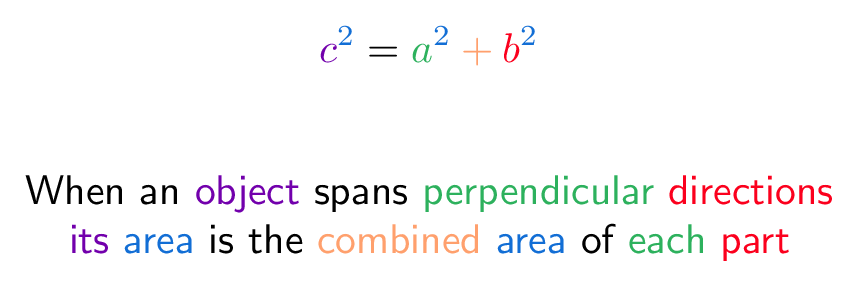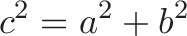# Pythagorean Theorem

## Colorized Definition\newcommand{\unified}{\color{c1}}
\newcommand{\partOne}{\color{c2}}
\newcommand{\partTwo}{\color{c3}}
\newcommand{\area}{\color{c4}}
\newcommand{\combined}{\color{c5}}$\displaystyle{\unified c^{\area 2} \plain = \partOne a^{\area 2} \combined + \partTwo b^{\area 2}}$

\plain     When an
\unified   object
\plain     spans
\partOne   perpendicular
\partTwo   directions
\
\unified   its
\area      area
\plain     is the
\combined  combined
\area      area
\plain     of
\partOne   each
\partTwo   part

## Read More - [How To Measure Any Distance With The Pythagorean Theorem](https://betterexplained.com/articles/measure-any-distance-with-the-pythagorean-theorem/) - [Surprising Uses of the Pythagorean Theorem](https://betterexplained.com/articles/surprising-uses-of-the-pythagorean-theorem/)